Question

Intense white light is incident on a diffraction grating that has 460 lines/mm.

(a) What is the highest order in which the complete visible spectrum can be seen with this grating? (Enter 1 for first order, 2 for second order, etc.)

(b) What is the angular separation between the violet edge (400 nm) and the red edge (700 nm) of the first order spectrum produced by the grating?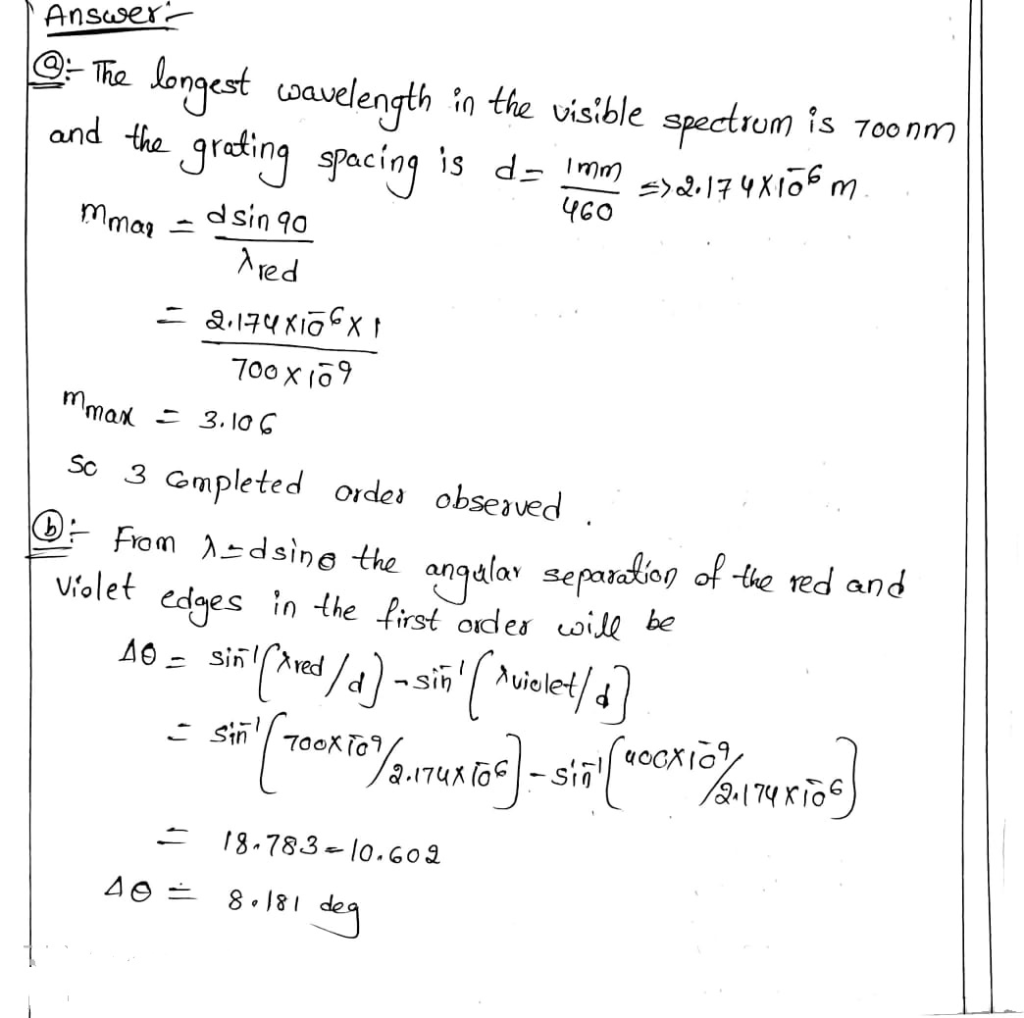#### Earn Coins

Coins can be redeemed for fabulous gifts.

Similar Homework Help Questions
• ### White light is normally incident on a diffraction grating that has 6500 lines/cm. What is the...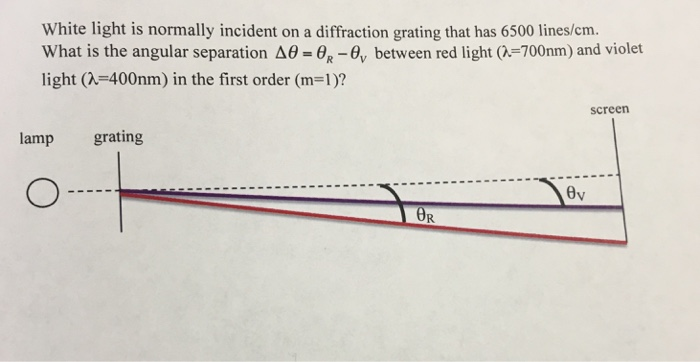White light is normally incident on a diffraction grating that has 6500 lines/cm. What is the angular separation Delta theta = theta_R - theta_V between red light (lambda = 700nm) and violet light (lambda = 400nm) in the first order (m = 1)?

• ### (a) White light is spread out into its spectral components by a diffraction grating. If the...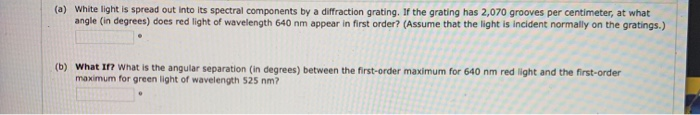(a) White light is spread out into its spectral components by a diffraction grating. If the grating has 2,070 grooves per centimeter, at what angle (in degrees) does red light of wavelength 640 nm appear in first order? (Assume that the light is incident normally on the gratings.) (b) What If? What is the angular separation (in degrees) between the first-order maximum for 640 nm red light and the first-order maximum for green light of wavelength 525 nm? 11. (-/1...

• ### A diffraction grating has 450 lines per mm. What is the highest order that contains the...

A diffraction grating has 450 lines per mm. What is the highest order that contains the entire visible spectrum from 400 nm to 700 nm? 2 5 6 3 4

• ### White light is made of all colours of the visible spectrum which has a wavelength range...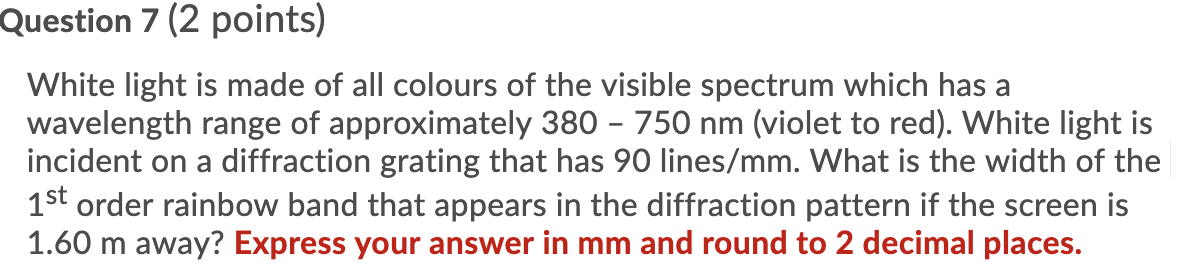White light is made of all colours of the visible spectrum which has a wavelength range of approximately 380 – 750 nm (violet to red). White light is incident on a diffraction grating that has 90 lines/mm. What is the width of the 1st order rainbow band that appears in the diffraction pattern if the screen is 1.60 m away? Express your answer in mm and round to 2 decimal places. Question 7 (2 points) White light is made of...

• ### White light is incident on a diffraction grating with 479 lines/mm. HINT (a) Calculate the angle...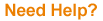White light is incident on a diffraction grating with 479 lines/mm. HINT (a) Calculate the angle θr2 (in degrees) to the second-order maximum for a wavelength of 620 nm. ° (b) Calculate the wavelength in nm of light with a third-order maximum at the same angle θr2. nm

• ### 28. When a narrow beam of white light is normally incident on a diffraction grating, you observe ...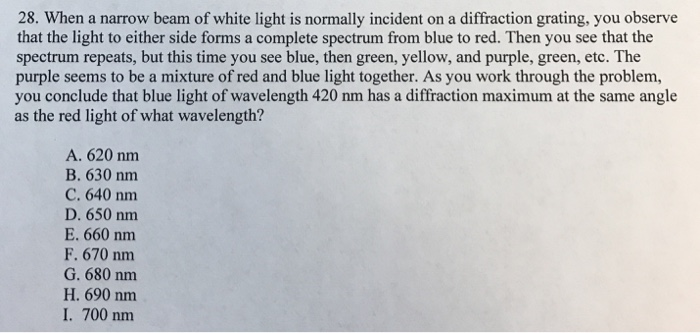28. When a narrow beam of white light is normally incident on a diffraction grating, you observe that the light to either side forms a complete spectrum from blue to red. Then you see that the spectrum repeats, but this time you see blue, then green, yellow, and purple, green, etc. The purple seems to be a mixture of red and blue light together. As you work through the problem, you conclude that blue light of wavelength 420 nm has...

• ### If a diffraction grating produces a third-order bright spot for red light of wavelength 700 nm, at 65° from the central maximum at what angle will the second order bright spot be for violet light...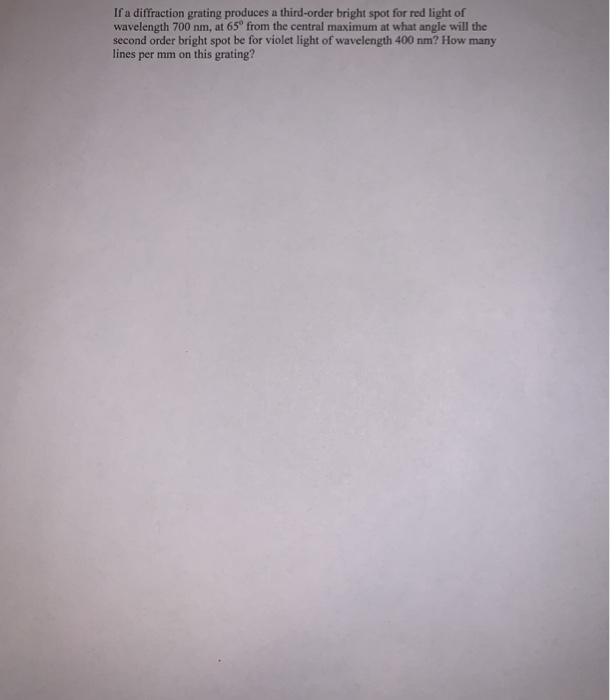If a diffraction grating produces a third-order bright spot for red light of wavelength 700 nm, at 65° from the central maximum at what angle will the second order bright spot be for violet light of wavelength 400 nm? How many lines per mm on this grating? If a diffraction grating produces a third-order bright spot for red light of wavelength 700 nm, at 65° from the central maximum at what angle will the second order bright spot be for...

• ### A diffraction grating having 180 lines/mm is illuminated with a light signal containing only two wavelengths,...

A diffraction grating having 180 lines/mm is illuminated with a light signal containing only two wavelengths, λ1 = 444 nm and λ2 = 555 nm. The signal is incident perpendicularly on the grating. (a) What is the angular separation between the second-order maxima of these two wavelengths? (b) What is the smallest angle at which two of the resulting maxima are superimposed? (c) What is the highest order for which maxima for both wavelengths are present in the diffraction pattern?

• ### White light is made of all colours of the visible spectrum which has a wavelength range...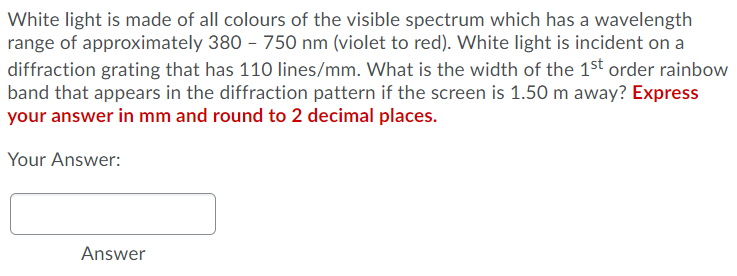White light is made of all colours of the visible spectrum which has a wavelength range of approximately 380 - 750 nm (violet to red). White light is incident on a diffraction grating that has 110 lines/mm. What is the width of the 1st order rainbow band that appears in the diffraction pattern if the screen is 1.50 m away? Express your answer in mm and round to 2 decimal places. Your Answer: Answer

• ### Sunlight is incident on a diffraction grating that has 3,350 lines/cm. The second-order spectrum over the...

Sunlight is incident on a diffraction grating that has 3,350 lines/cm. The second-order spectrum over the visible range (400-700 nm) is to be limited to 1.50 cm along a screen that is a distance L from the grating. What is the required value of L? [answer] cm